# 15-344/Homework Assignment 7

Jump to: navigation, search

This assignment is due at the tutorials on Thursday November 19. Here and everywhere, neatness counts!! You may be brilliant and you may mean just the right things, but if the teaching assistants will be having hard time deciphering your work they will give up and assume it is wrong.

Reread sections 5.1-5.4 of our textbook. Remember that reading math isn't like reading a novel! If you read a novel and miss a few details most likely you'll still understand the novel. But if you miss a few details in a math text, often you'll miss everything that follows. So reading math takes reading and rereading and rerereading and a lot of thought about what you've read. Also, preread sections 5.5 and 6.1, just to get a feel for the future.

Solve problems 8, 10, 15, 20, 24, 28, and 29 in section 5.3 and problems 1, 3, 16, 39, 40, 63, and 65 in section 5.4, but submit only your solutions of the underlined problems.

 Dror's notes above / Students' notes below

In problem 29, Section 5.3, we were asked to prove that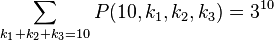$\sum_{k_1 + k_2 + k_3 = 10} P(10, k_1, k_2, k_3) = 3^{10}$, where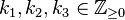$k_1, k_2, k_3 \in \mathbb{Z}_{\geq 0}$.

It is tempting to think that this is a special case of some identity and that a general pattern can be found.

Replacing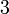$3$ with any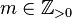$m \in \mathbb{Z}_{>0}$ and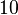$10$ with any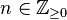$n \in \mathbb{Z}_{\geq0}$ gives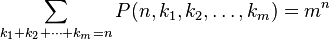$\sum_{k_1+k_2+\cdots+k_m=n} P(n, k_1, k_2, \ldots, k_m) = m^n$

Does it hold, or can it be generalized to a greater extent?

Yes. We have the Multinomial_theorem.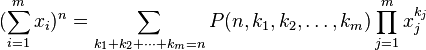$(\sum_{i=1}^m x_i)^n = \sum_{k_1 + k_2 + \cdots + k_m = n} P(n, k_1, k_2, \ldots, k_m) \prod_{j=1}^m x_{j}^{k_{j}}$, where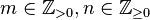$m \in \mathbb{Z}_{>0}, n \in \mathbb{Z}_{\geq0}$ and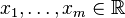$x_1, \ldots, x_m\in \mathbb{R}$.

For a proof by induction, see here.